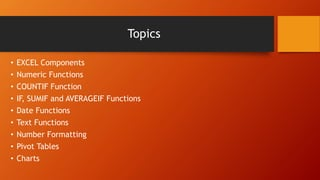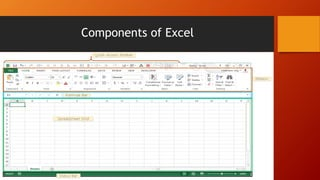Diese Präsentation wurde erfolgreich gemeldet.

# Introduction to MS Excel

Anzeige
Anzeige
Anzeige
Anzeige
Anzeige
Anzeige
Anzeige
Anzeige
Anzeige
Anzeige
Anzeige×

1 von 19 Anzeige

# Introduction to MS Excel

Basic Excel training: the training discussed the main components of excel, numeric functions and how to run arithmetic calculations in excel, date functions, text functions, boolean functions, pivot tables, and charts.

Basic Excel training: the training discussed the main components of excel, numeric functions and how to run arithmetic calculations in excel, date functions, text functions, boolean functions, pivot tables, and charts.

Anzeige
Anzeige

### Introduction to MS Excel

1. 1. Introduction to Excel Training Tarek Dib Monitoring and Evaluation Consultant, AMURT Beirut, Lebanon Phone: 03/046829 Emails: tdib@amurt.email OR tdib03@gmail.com
2. 2. Topics • EXCEL Components • Numeric Functions • COUNTIF Function • IF, SUMIF and AVERAGEIF Functions • Date Functions • Text Functions • Number Formatting • Pivot Tables • Charts
3. 3. Components of Excel
4. 4. Numeric Functions • Numeric functions in Excel develop computation on numbers • Some of these functions would be to find the sum of numbers, average, smallest or largest number, median, count, etc. • Average of numbers is the sum of all numbers divided by how many numbers there are in a range of cells. Example: Assume that the Weights in Kg of 10 people are: 70, 60, 55, 45, 65, 65, 55, 85, 40 and 90. Then the average weight = (70 + 60 + 55 + 45 + 65 + 65 + 55 + 85 + 40 + 90)/10 = 63. However, in Excel, you may use the AVERAGE() function instead. • Median is the 50th percentile of a set of numbers. In other word, it is the middle of a set of numbers. If we take the same weight example above. Manually, it is computed as follows: first you sort the data in ascending order i.e. from smallest to largest, and you take the middle number or numbers. Sorted data: 40, 45, 55, 55, 60, 65, 65, 70, 85, 90. Median = (60 + 65)/2 = 62.5. In excel you may use the MEDIAN() function instead.
5. 5. Numeric Functions - Continued • SUM(): Finds the sum of numbers in a range of cells • MIN(): Returns the smallest number in a range of cells • MAX(): Returns the largest number in a range of cells • MODE(): Returns the most frequent i.e. repeated number • COUNT(): It counts how many cells in a given range contains numbers • COUNTA(): It count the number of cells in a given range that contain characters such as numbers, text, or symbols • SQRT(): Finds the square root of a number
6. 6. Numeric Functions - Examples
7. 7. The COUNT() Function Family • COUNT() and COUNTA() have been defined previously in Slide 5 • COUNTIF(): is a function to count cells that meet a single criteria. This is illustrated in the example below Another example is use the weight data to find how many weigh above the average i.e. above 63 Kg. The formula to be used is: COUNTIF(B2:B11,">=63")
8. 8. IF(), SUMIF() and AVERAGEIF() Functions • IF() function: The IF() function returns one value for a TRUE result, and another for a FALSE result • The formula for IF() function is “=IF (logical_test, [value_if_true], [value_if_false])”. It will clear when we use the example in the next slide • SUMIF() function is used to sum the amount which meets specific criteria • The formula of SUMIF() is “=SUMIF(range,criteria,sum_range)” • AVERAGEIF() function is similar to SUMIF(), but finds the average instead
9. 9. IF(), SUMIF() and AVERAGEIF() Functions - Examples
10. 10. Date Functions Date functions that we will look at in this training are the following: • Day(): Returns an integer between 1 and 31 • Weekday(): Returns an integer between 1 and 7 (1: Monday, 2: Tuesday, up to 7: Sunday) • Year(): Returns the year in a certain date • Today(): Returns the current date without the timestamp • Now(): Same as Today() but returns the time • Datedif(): Returns the period in years, days, etc. between two dates • Days(): Returns the number of days between two dates • Text(): Returns the day of the week (Monday through Sunday) (Text(date, “dddd”) to return the day of the week
11. 11. Date Functions - Examples
12. 12. Text Functions
13. 13. Pivot Table • Pivot table is an effective tool in Excel to group data by categories such as gender, nationality, age group, etc. • For this example, we will create a pivot table to count the number of Lebanese and Syrian households in each work type. Steps to create this pivot table are: 1. Select the table or cells (including column headers) you want to include in your PivotTable
14. 14. Pivot Table - Continued 2. From the Insert tab, click the PivotTable command 3. The Create PivotTable dialog box will appear. Choose your settings, then click OK. In our example, we'll use Table1 as our source data and place the PivotTable on a new worksheet
15. 15. Pivot Table - Continued 4. A blank PivotTable and Field List will appear on a new worksheet
16. 16. Pivot Table - Continued 5. Once you create a PivotTable, you'll need to decide which fields to add. Each field is simply a column header from the source data. In the PivotTable Field List, check the box for each field you want to add. In our example, we want to know the number of Syrians and Lebanese in each work type. We will check Nationality and work_type
17. 17. Charts/ Graphs with Example 1. Highlight the range of data with the column header included 2. Go to Insert Tab, and under the Charts, select the type of chart or Recommended Charts window to select the appropriate chart you would like to have in your report 3. In the example, we are having Days of the week and the run time in minutes each day. For such data fields, the best charts would either be the bar/ column or line chart since the x-axis is a categorical variable and the y-axis is a numerical variable. There are other types of charts that you shall explore in your own
18. 18. Charts/ Graphs with Example – Column vs. Line Chart 0 10 20 30 40 50 60 70 80 90 100 Mon Tue Wed Thu Fri Sat Sun TIMEINMINUTES DAY Daily Run Time April 16th to 22nd 33 0 32 86 50 76 0 MON TUE WED THU FRI SAT SUN TimeinMinutes Day Daily Run Time April 16th to 22nd
19. 19. Thank you. Hope to see you in the near future. Until then, remember that it is not worth stressing about things we can’t change. The only control I can have upon is me and myself. Happiness is an inner job, and as I said no one is responsible of neither my happiness nor my misery. Also remember to go out and explore what this earth has gifted us with plenty of love and beauty!